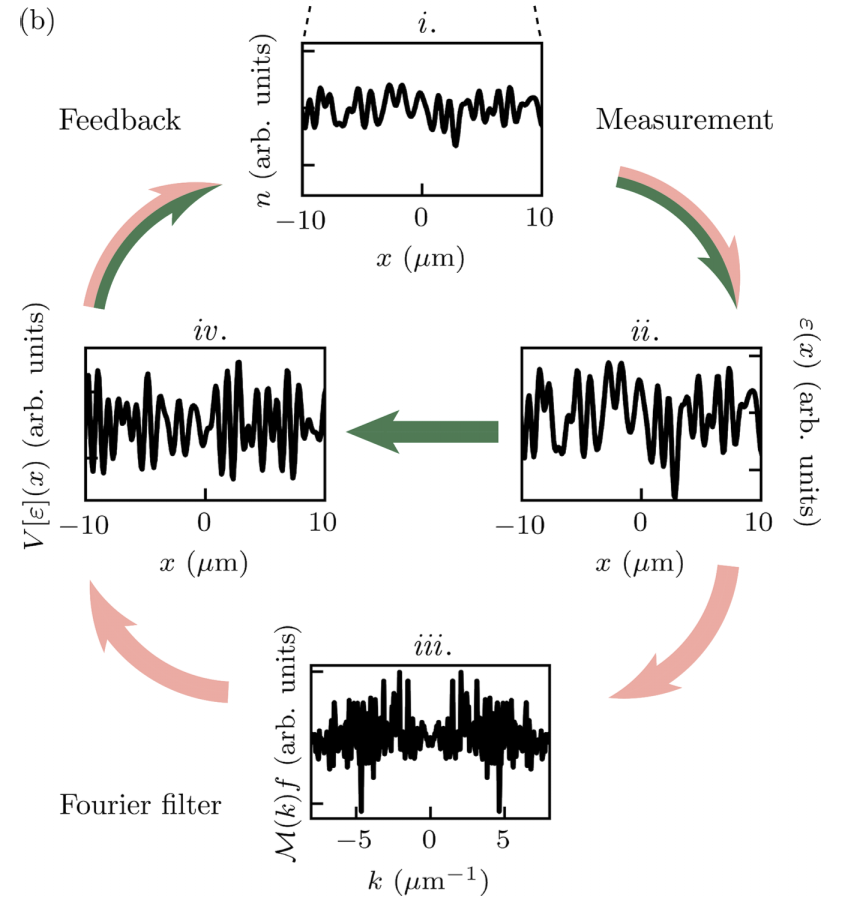# Feedback-cooled Bose-Einstein condensation: Near and far from equilibriumContinuously measured interacting quantum systems almost invariably heat, causing loss of quantum coherence. Here, we study Bose-Einstein condensates (BECs) subject to repeated weak measurement of the atomic density and describe several protocols for generating a feedback signal designed to remove excitations created by measurement back-action. We use a stochastic Gross-Pitaevskii equation to model the system dynamics and find that a feedback protocol utilizing momentum-dependent gain and filtering can effectively cool both one-dimensional (1D) and two-dimensional systems. The performance of these protocols is quantified in terms of the steady-state energy, entropy, and condensed fraction. These are the first feedback cooling protocols demonstrated in two dimensions, and in one dimension our optimal protocol reduces the equilibrium energy by more than a factor of 100 as compared with a previous cooling protocol developed using the same methodology. We also use this protocol to quench-cool 1D BECs from noncondensed highly excited states and find that they rapidly condense into a far-from-equilibrium state with energy orders of magnitude higher than the equilibrium ground-state energy for that condensate fraction. We explain this in terms of the near integrability of our 1D system, whereby efficiently cooled low-momentum modes are effectively decoupled from the energetic “reservoir” of the higher-momentum modes. We observe that the quench-cooled condensed states can have nonzero integer winding numbers described by quantized supercurrents.

Feedback-cooled Bose-Einstein condensation: Near and far from equilibrium; E. P. Yamaguchi, H. M. Hurst, and I. B. Spielman; Phys. Rev. A 107 063306 (2023). doi:10.1103/PhysRevA.107.063306